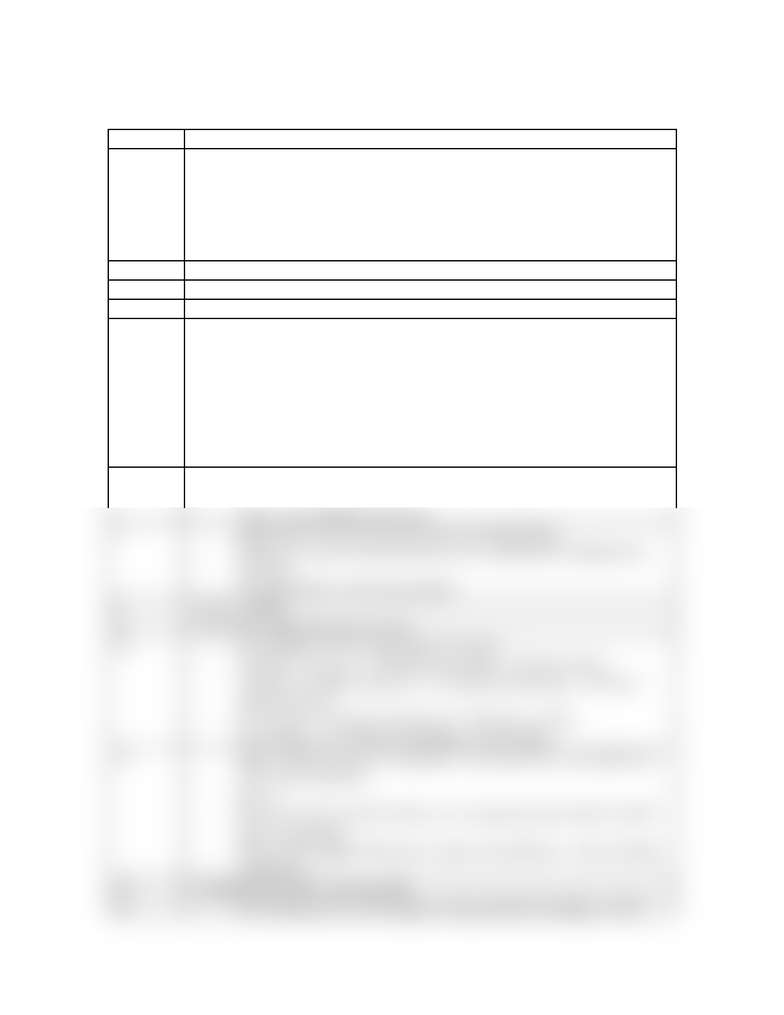# Notes taken during lecture

35 views2 pages
School
Department
Course
ProfessorLECTURE 6 – Solutions
Slide No. Notes
1-dilute – not much solute
-concentrated – lots of solute
-saturated – maximum amount of solute dissolved
-unsaturated – less than maximum amount of solute dissolved
-supersaturated – produced by heating the solution, dissolving more
solute, then letting it cool
2- molality is independent of temperature
3- 1.45g (mol/342.3g) / (30.0ml)(1.00g/mol)(kg/1000g) = 0.141 mol/kg
4- miscible = can be mixed together in any proportions
5-network solids – ex. diamonds – insoluble in all solvents
-metals – insoluble in liquid solvents; can dissolve in other metals
-ex. brass = zinc and copper
-ionic solids – will dissolve if solvent has dipole-ion interactions
strong enough
-molecular solids – will dissolve in solvent with same type of
intermolecular forces
-ex. glucose can H-bond with water
6-Ans1: C
-Ans2: B – depends on substance
-Ans3 – gas solubility decreases
7-pushing down forces gas molecules into liquid phase
-higher rate of gas dissolving means more liquid than changing into
gas phase
-rate dissolving = rate into gas phase
8- Torr = mmHg
9- (a) to (b), vapour pressure is lower
12 -P of solution = X of water times P of water
-100.0mL of water = (1.00g/mL)(mol/18g) = 5.56 mol water
-100.0mL of ethylene glycol = (1.15g/mL)(mol/62.0g) = 1.86 mol
ethylene glycol
-X of water = 5.56 mol / (5.56 mol + 1.86 mol) = 0.749
-P of solution = (0.749)(525.8mmHg) = 394 mmHg
13 -higher vapour pressure if separated – B because less concentrated so
more of B evaporates
-Ans: A
-Because in the closed box there is no vapour pressure until A and B
starts evaporating
-Both will be happy if they have equal concentrations – thus reaching
equilibrium
14 - adding more sucrose increases BP
15 -Tb = kb times m = (2.53 degrees C kg/ mol)(0.10 mol/kg) = 0.253
www.notesolution.com
Unlock document

This preview shows half of the first page of the document.
Unlock all 2 pages and 3 million more documents.

## Document Summary

1. 45g (mol/342. 3g) / (30. 0ml)(1. 00g/mol)(kg/1000g) = 0. 141 mol/kg. Miscible = can be mixed together in any proportions network solids ex. diamonds insoluble in all solvents. Metals insoluble in liquid solvents; can dissolve in other metals ex. brass = zinc and copper ionic solids will dissolve if solvent has dipole-ion interactions strong enough. Molecular solids will dissolve in solvent with same type of intermolecular forces ex. glucose can h-bond with water. Ans3 gas solubility decreases pushing down forces gas molecules into liquid phase higher rate of gas dissolving means more liquid than changing into gas phase rate dissolving = rate into gas phase. (a) to (b), vapour pressure is lower. P of solution = x of water times p of water. 100. 0ml of water = (1. 00g/ml)(mol/18g) = 5. 56 mol water. 100. 0ml of ethylene glycol = (1. 15g/ml)(mol/62. 0g) = 1. 86 mol ethylene glycol. X of water = 5. 56 mol / (5. 56 mol + 1. 86 mol) = 0. 749.

# Get access

\$10 USD/m
Billed \$120 USD annually
Homework Help
Class Notes
Textbook Notes